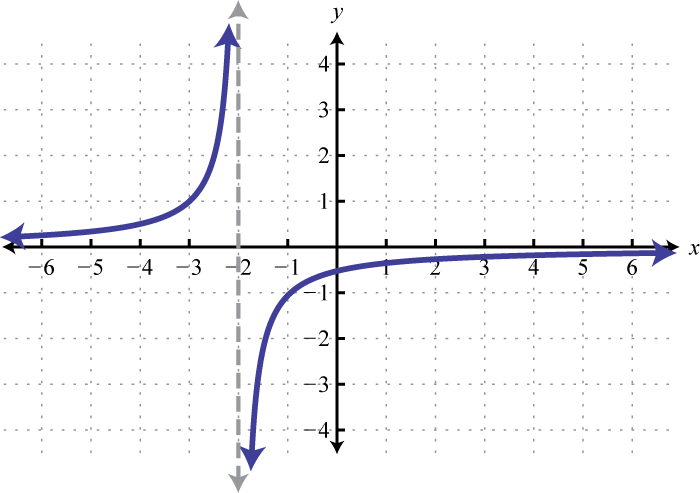## 2.5 Using Transformations to Graph Functions

### Learning Objectives

1. Define the rigid transformations and use them to sketch graphs.
2. Define the non-rigid transformations and use them to sketch graphs.

## Vertical and Horizontal Translations

When the graph of a function is changed in appearance and/or location we call it a transformation. There are two types of transformations. A rigid transformationA set of operations that change the location of a graph in a coordinate plane but leave the size and shape unchanged. changes the location of the function in a coordinate plane, but leaves the size and shape of the graph unchanged. A non-rigid transformationA set of operations that change the size and/or shape of a graph in a coordinate plane. changes the size and/or shape of the graph.

A vertical translationA rigid transformation that shifts a graph up or down. is a rigid transformation that shifts a graph up or down relative to the original graph. This occurs when a constant is added to any function. If we add a positive constant to each y-coordinate, the graph will shift up. If we add a negative constant, the graph will shift down. For example, consider the functions $g(x)=x2−3$ and $h(x)=x2+3.$ Begin by evaluating for some values of the independent variable x.Now plot the points and compare the graphs of the functions g and h to the basic graph of $f(x)=x2$, which is shown using a dashed grey curve below.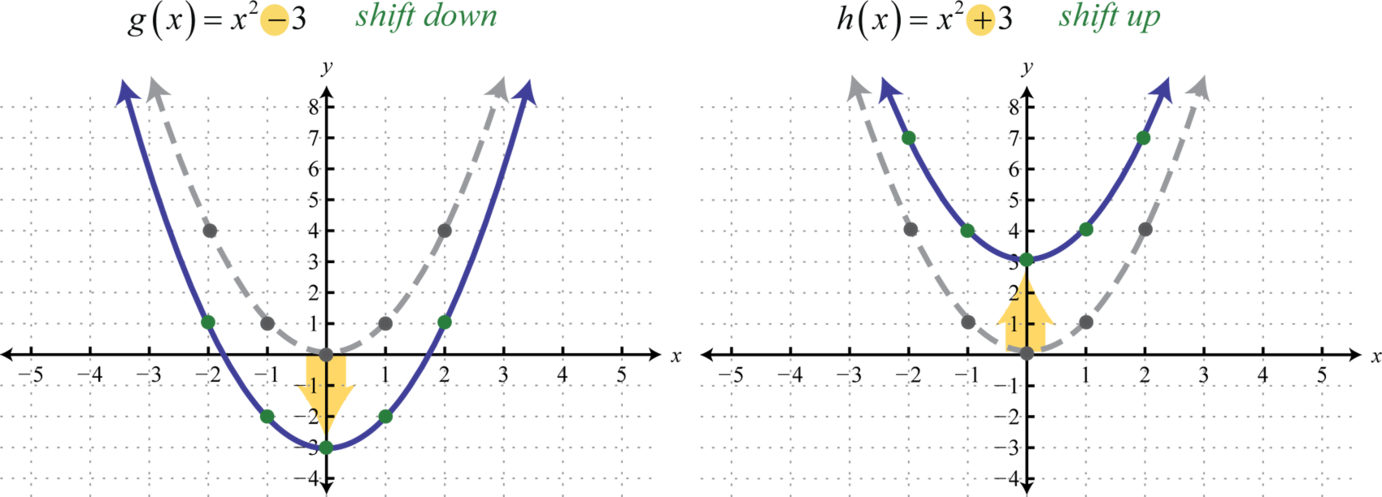The function g shifts the basic graph down 3 units and the function h shifts the basic graph up 3 units. In general, this describes the vertical translations; if k is any positive real number:

 Vertical shift up k units: $F(x)=f(x)+k$ Vertical shift down k units: $F(x)=f(x)−k$

### Example 1

Sketch the graph of $g(x)=x+4.$

Solution:

Begin with the basic function defined by $f(x)=x$ and shift the graph up 4 units.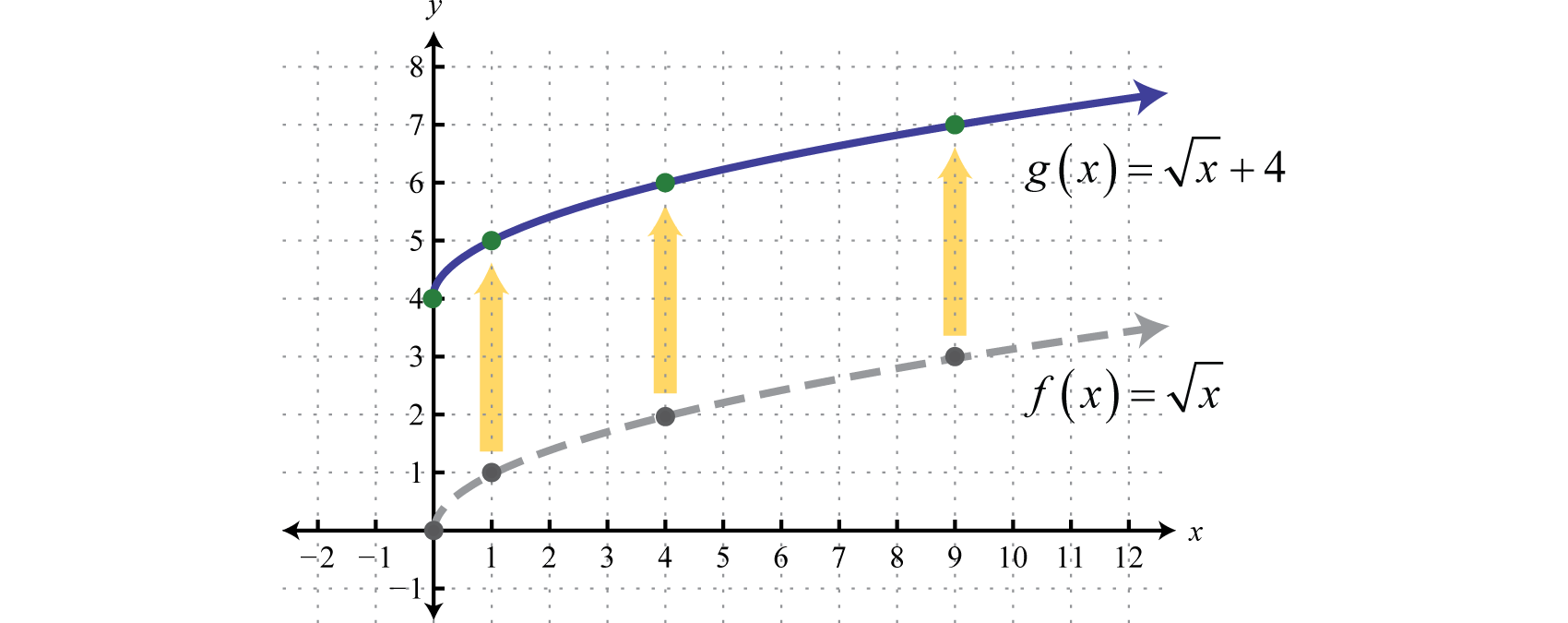A horizontal translationA rigid transformation that shifts a graph left or right. is a rigid transformation that shifts a graph left or right relative to the original graph. This occurs when we add or subtract constants from the x-coordinate before the function is applied. For example, consider the functions defined by $g(x)=(x+3)2$ and $h(x)=(x−3)2$ and create the following tables: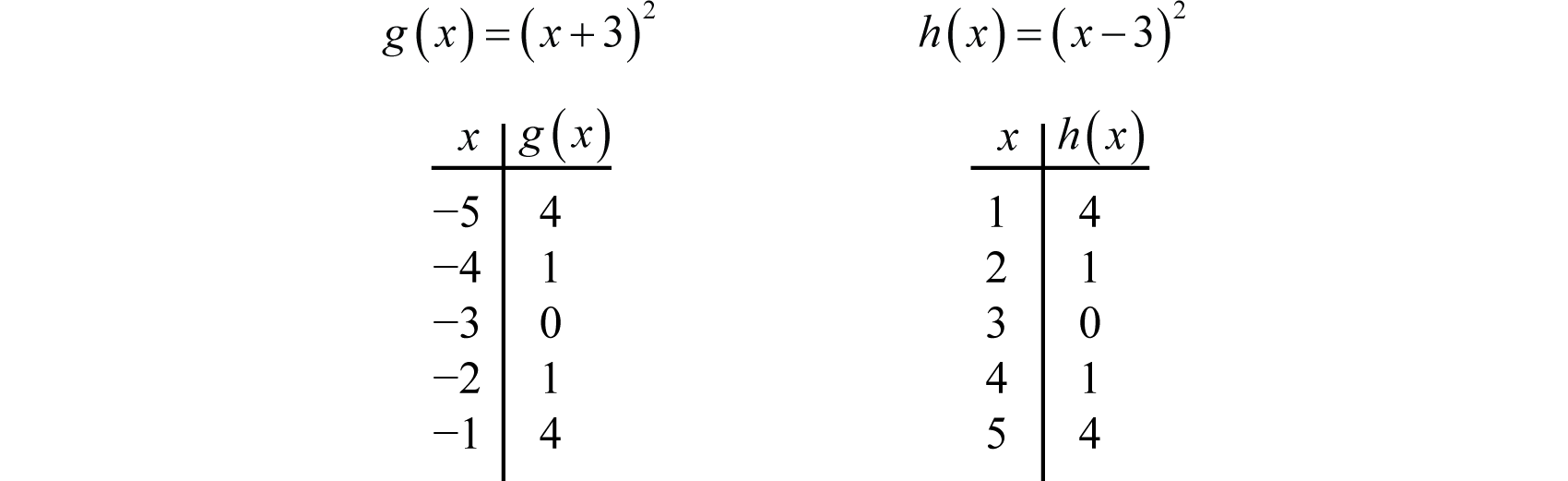Here we add and subtract from the x-coordinates and then square the result. This produces a horizontal translation.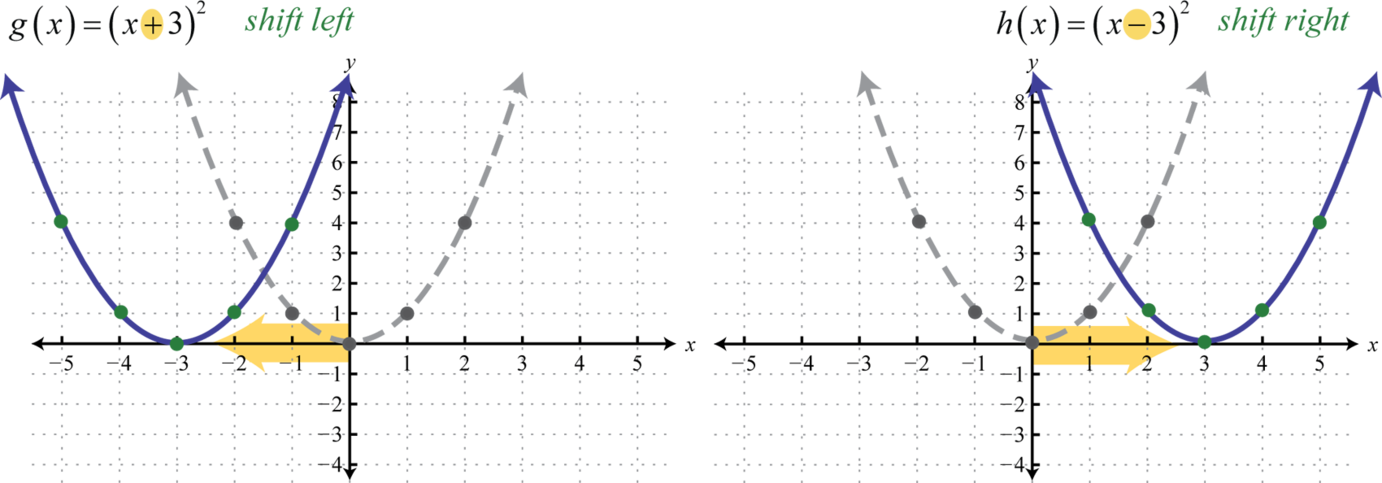Note that this is the opposite of what you might expect. In general, this describes the horizontal translations; if h is any positive real number:

 Horizontal shift left h units: $F(x)=f(x+h)$ Horizontal shift right h units: $F(x)=f(x−h)$

### Example 2

Sketch the graph of $g(x)=(x−4)3.$

Solution:

Begin with a basic cubing function defined by $f(x)=x3$ and shift the graph 4 units to the right.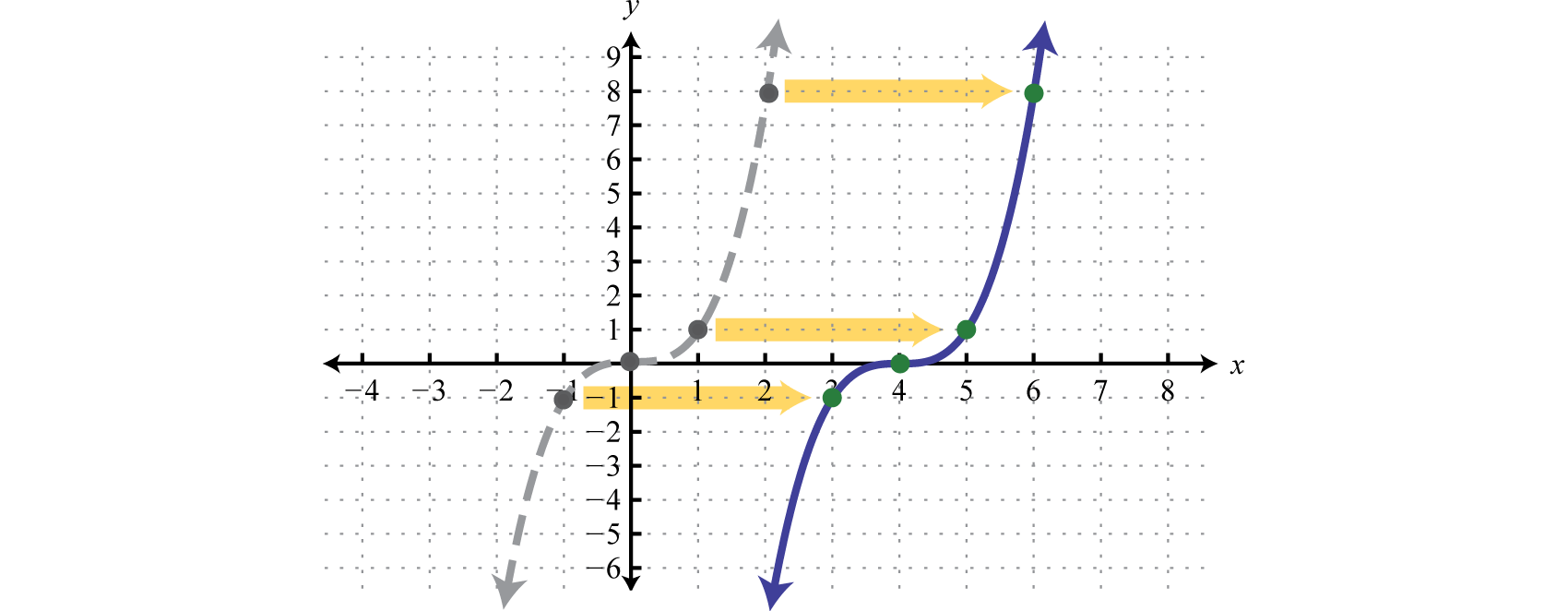It is often the case that combinations of translations occur.

### Example 3

Sketch the graph of $g(x)=|x+3|−5.$

Solution:

$y=|x|Basic functiony=|x+3|Horizontal shift left 3 unitsy=|x+3|−5Vertical shift down 5 units$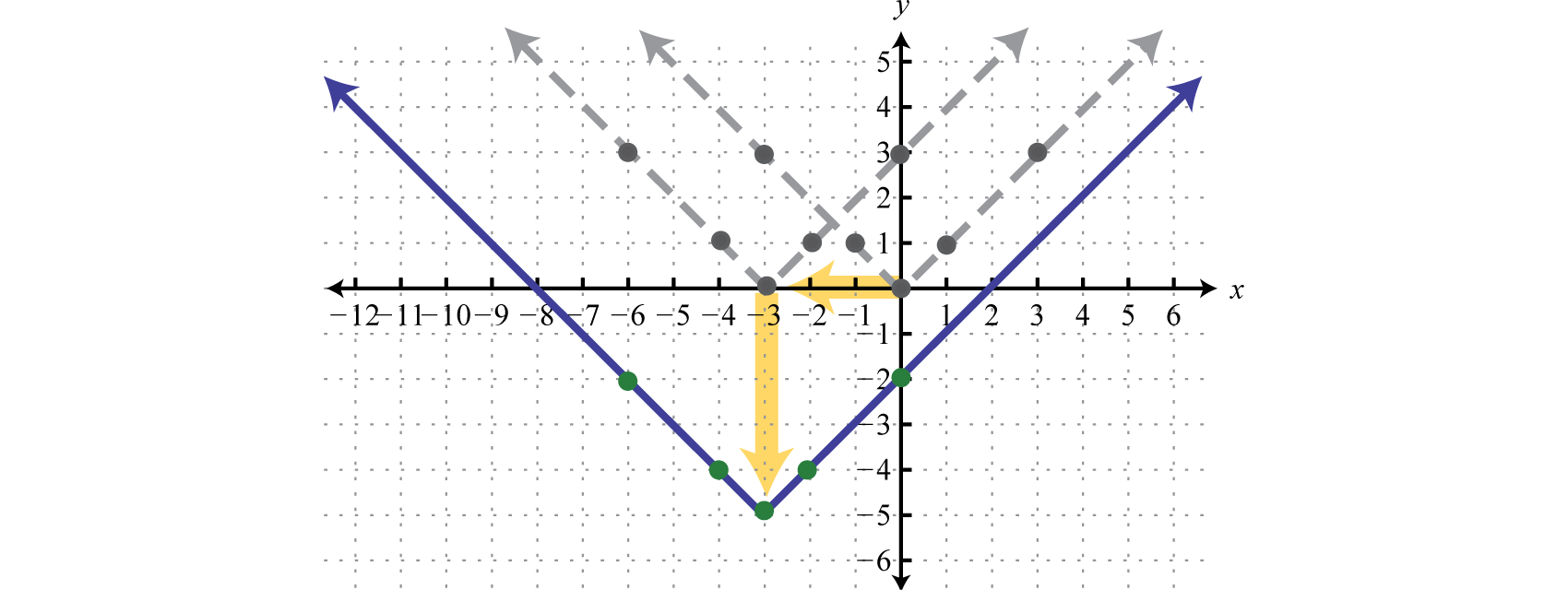The order in which we apply horizontal and vertical translations does not affect the final graph.

### Example 4

Sketch the graph of $g(x)=1x−5+3.$

Solution:

Begin with the reciprocal function and identify the translations.

$y=1xBasic functiony=1x−5Horizontal shift right 5 unitsy=1x−5+3Vertical shift up 3 units$

Take care to shift the vertical asymptote from the y-axis 5 units to the right and shift the horizontal asymptote from the x-axis up 3 units.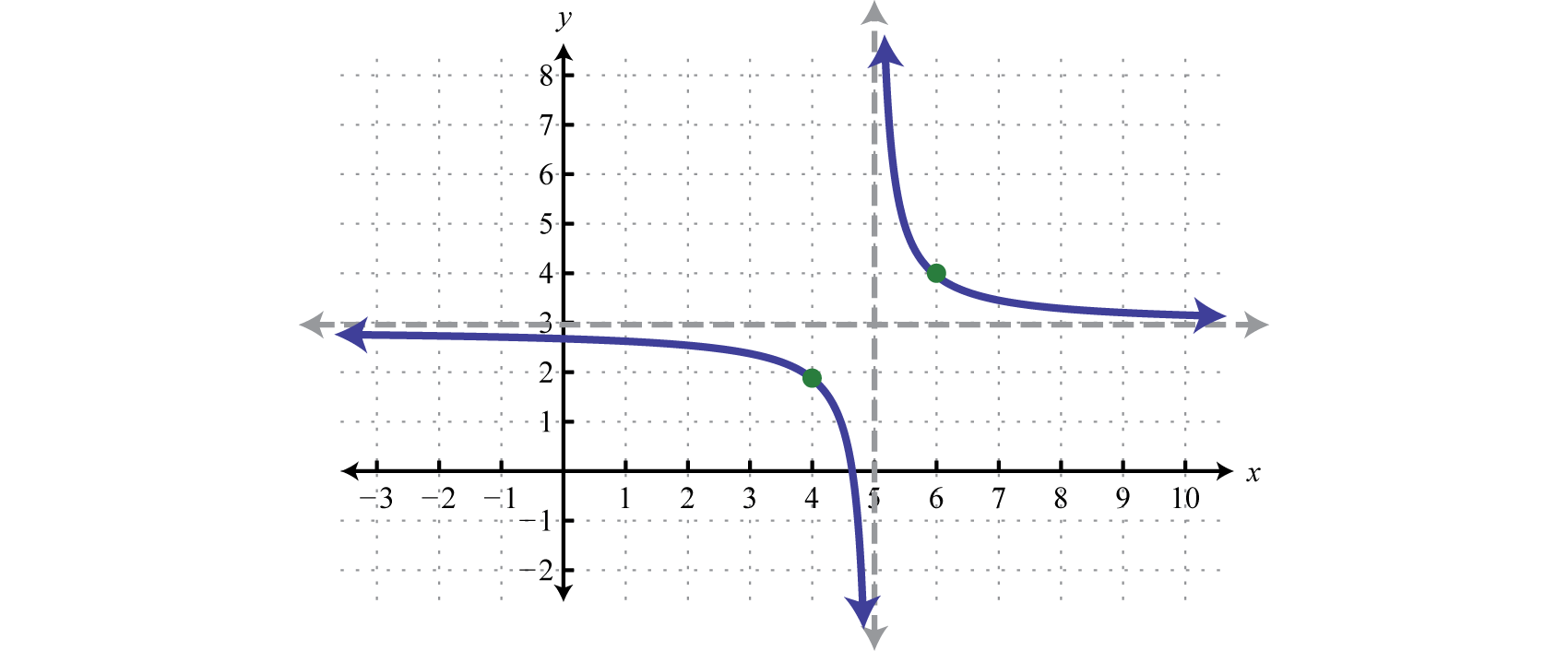Try this! Sketch the graph of $g(x)=(x−2)2+1.$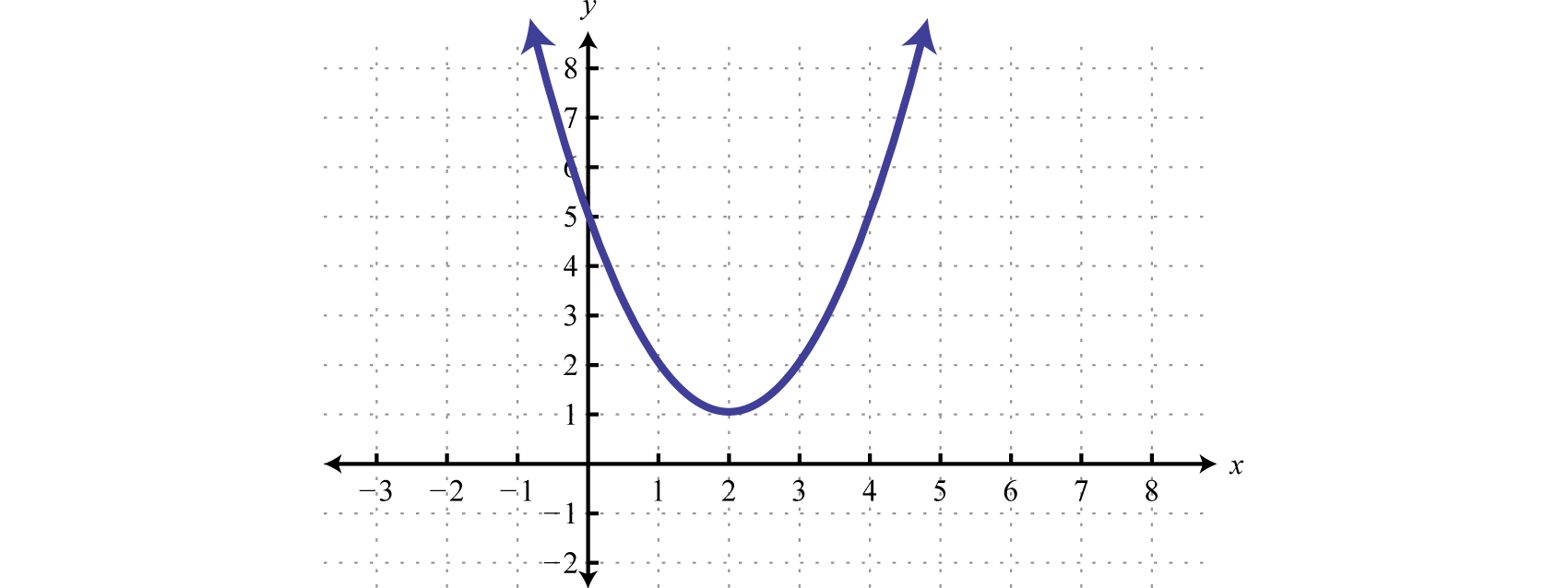## Reflections

A reflectionA transformation that produces a mirror image of the graph about an axis. is a transformation in which a mirror image of the graph is produced about an axis. In this section, we will consider reflections about the x- and y-axis. The graph of a function is reflected about the x-axis if each y-coordinate is multiplied by −1. The graph of a function is reflected about the y-axis if each x-coordinate is multiplied by −1 before the function is applied. For example, consider $g(x)=−x$ and $h(x)=−x.$Compare the graph of g and h to the basic square root function defined by $f(x)=x$, shown dashed in grey below: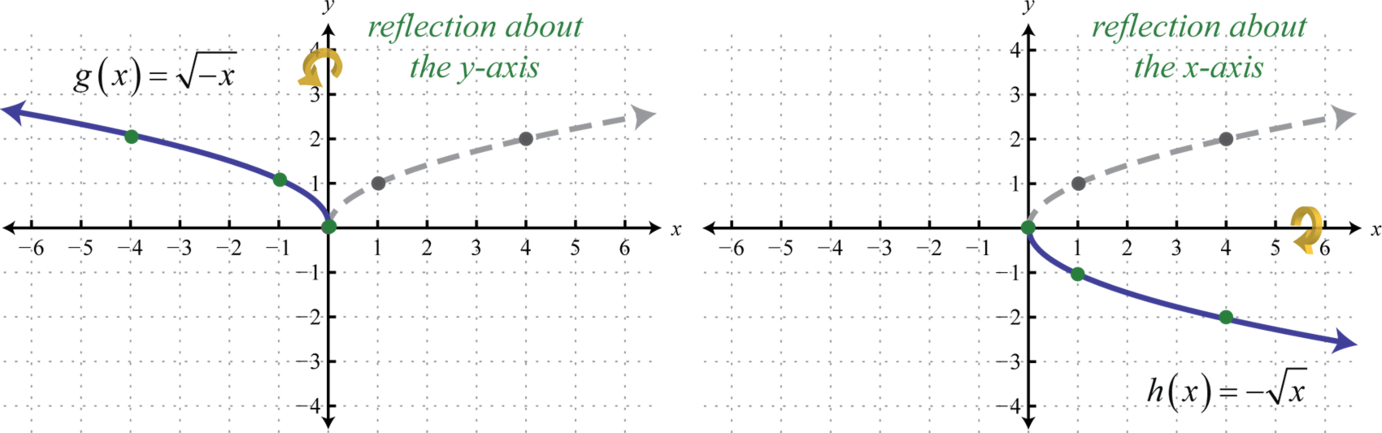The first function g has a negative factor that appears “inside” the function; this produces a reflection about the y-axis. The second function h has a negative factor that appears “outside” the function; this produces a reflection about the x-axis. In general, it is true that:

 Reflection about the y-axis: $F(x)=f(−x)$ Reflection about the x-axis: $F(x)=−f(x)$

When sketching graphs that involve a reflection, consider the reflection first and then apply the vertical and/or horizontal translations.

### Example 5

Sketch the graph of $g(x)=−(x+5)2+3.$

Solution:

Begin with the squaring function and then identify the transformations starting with any reflections.

$y=x2Basic function.y=−x2Reflection about the x-axis.y=−(x+5)2Horizontal shift left 5 units.y=−(x+5)2+3Vertical shift up 3 units.$

Use these translations to sketch the graph.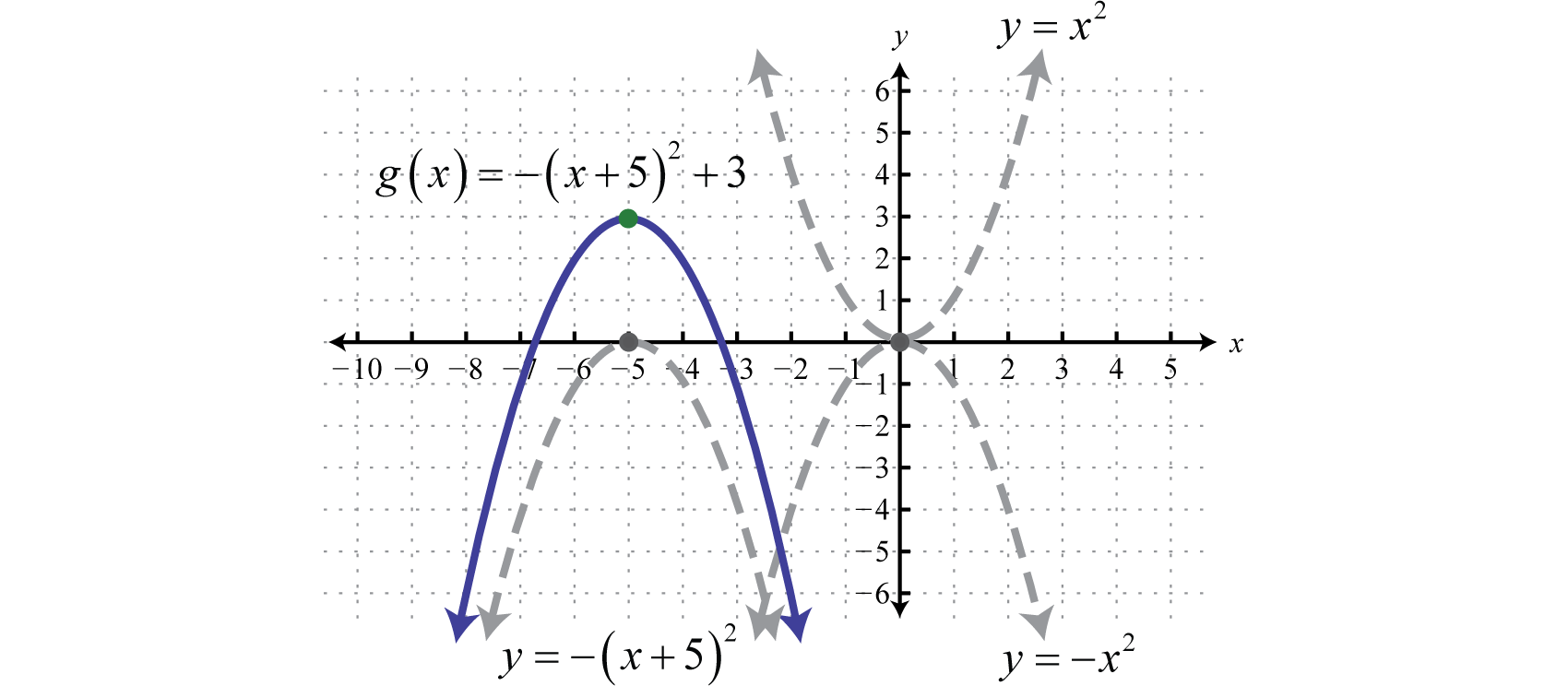Try this! Sketch the graph of $g(x)=−|x|+3.$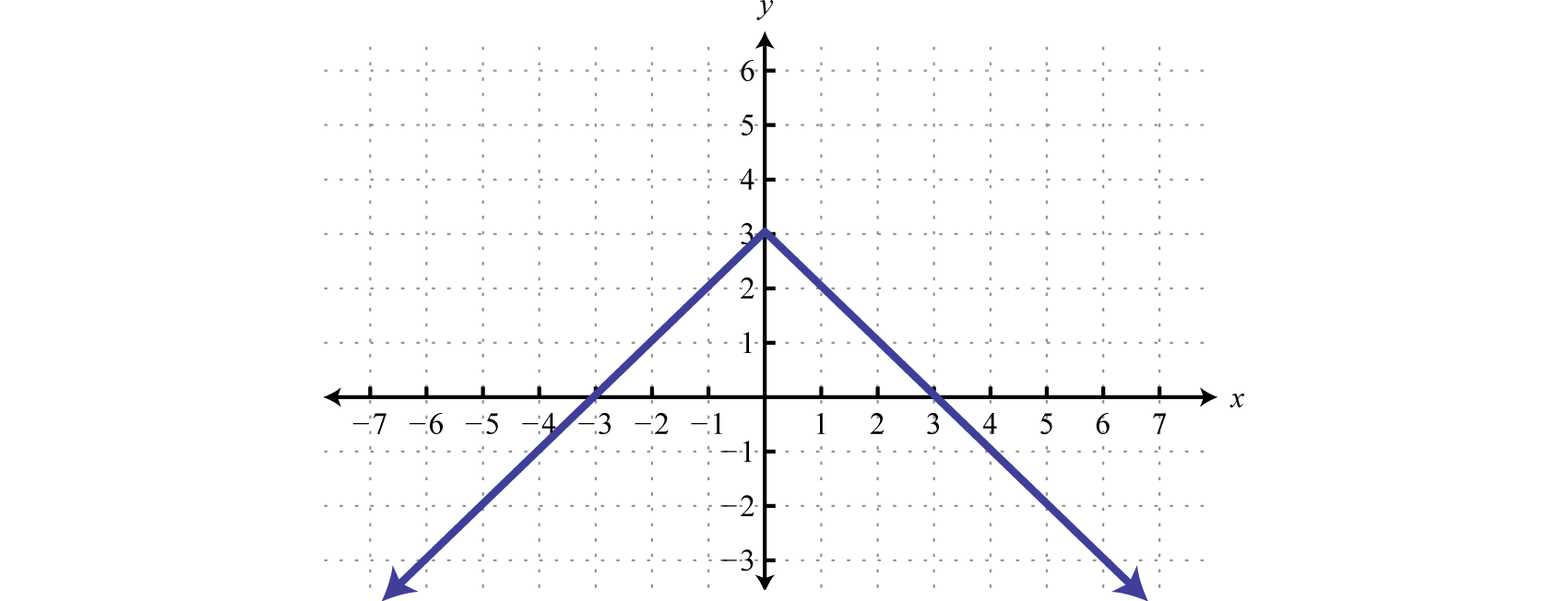## Dilations

Horizontal and vertical translations, as well as reflections, are called rigid transformations because the shape of the basic graph is left unchanged, or rigid. Functions that are multiplied by a real number other than 1, depending on the real number, appear to be stretched vertically or stretched horizontally. This type of non-rigid transformation is called a dilationA non-rigid transformation, produced by multiplying functions by a nonzero real number, which appears to stretch the graph either vertically or horizontally.. For example, we can multiply the squaring function $f(x)=x2$ by 4 and $14$ to see what happens to the graph.Compare the graph of g and h to the basic squaring function defined by $f(x)=x2$, shown dashed in grey below: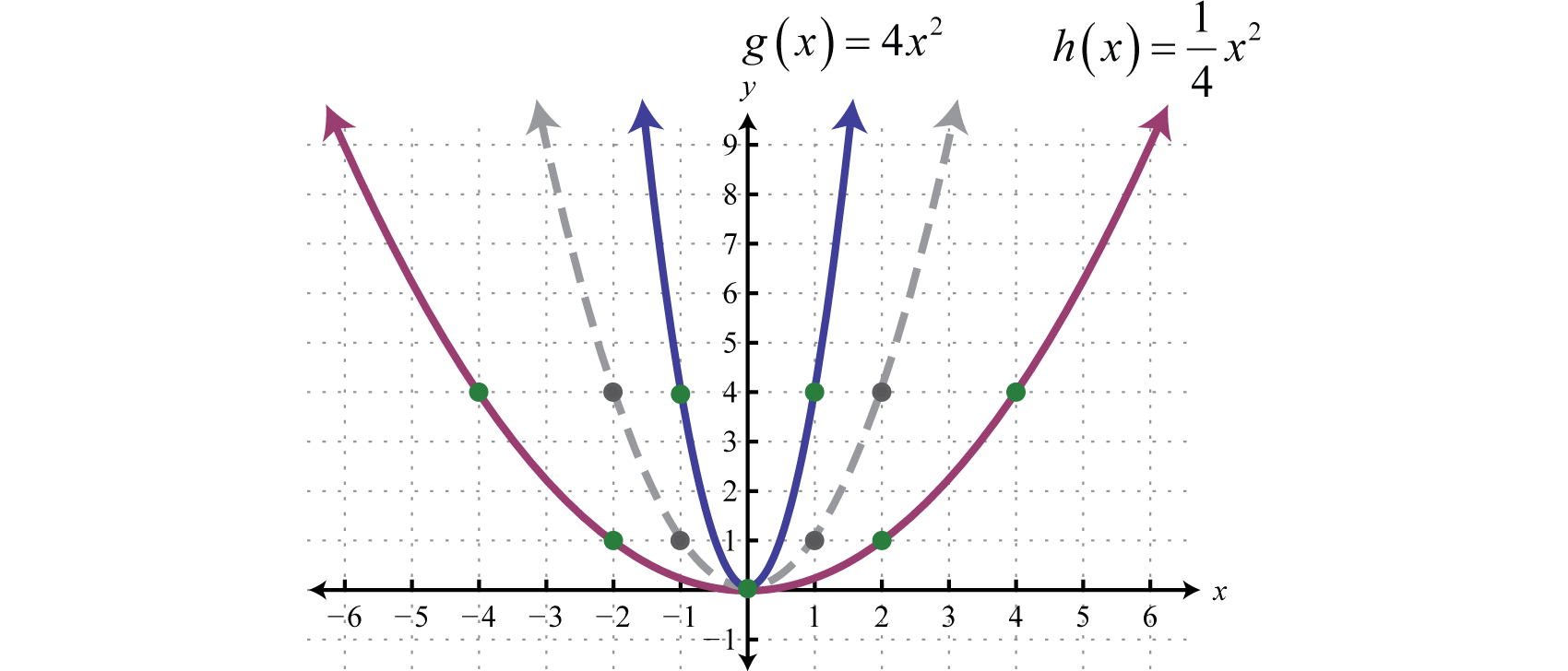The function g is steeper than the basic squaring function and its graph appears to have been stretched vertically. The function h is not as steep as the basic squaring function and appears to have been stretched horizontally.

In general, we have:

 Dilation: $F(x)=a⋅f(x)$

If the factor a is a nonzero fraction between −1 and 1, it will stretch the graph horizontally. Otherwise, the graph will be stretched vertically. If the factor a is negative, then it will produce a reflection as well.

### Example 6

Sketch the graph of $g(x)=−2|x−5|−3.$

Solution:

Here we begin with the product of −2 and the basic absolute value function: $y=−2|x|.$ This results in a reflection and a dilation.

$xyy=−2|x| ←Dilation and reflection−1−2y=−2|−1|=−2⋅1=−200y=−2|0|=−2⋅0=01−2y=−2|1|=−2⋅1=−2$

Use the points {(−1, −2), (0, 0), (1, −2)} to graph the reflected and dilated function $y=−2|x|.$ Then translate this graph 5 units to the right and 3 units down.

$y=−2|x|Basic graph with dilation andreflection about the x−axis.y=−2|x−5|Shift right 5 units.y=−2|x−5|−3Shift down 3 units.$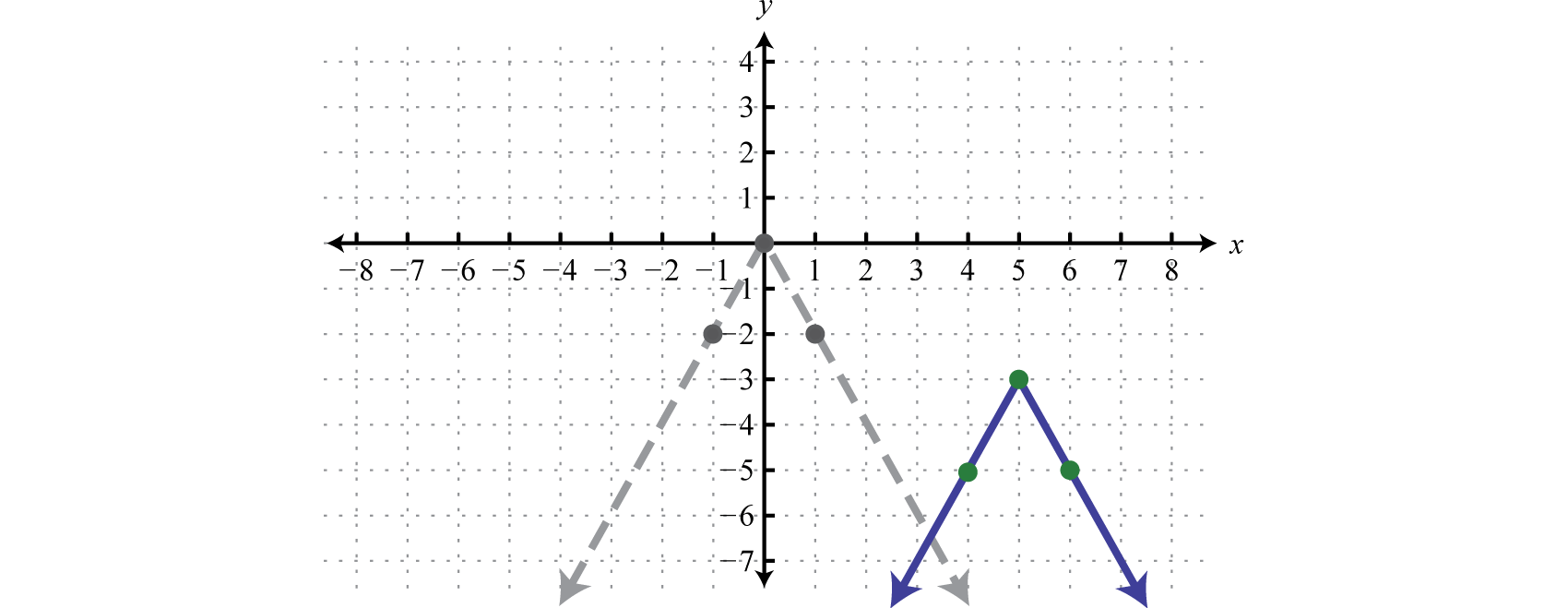In summary, given positive real numbers h and k:

 Vertical shift up k units: $F(x)=f(x)+k$ Vertical shift down k units: $F(x)=f(x)−k$

 Horizontal shift left h units: $F(x)=f(x+h)$ Horizontal shift right h units: $F(x)=f(x−h)$

 Reflection about the y-axis: $F(x)=f(−x)$ Reflection about the x-axis: $F(x)=−f(x)$

 Dilation: $F(x)=a⋅f(x)$

### Key Takeaways

• Identifying transformations allows us to quickly sketch the graph of functions. This skill will be useful as we progress in our study of mathematics. Often a geometric understanding of a problem will lead to a more elegant solution.
• If a positive constant is added to a function, $f(x)+k$, the graph will shift up. If a positive constant is subtracted from a function, $f(x)−k$, the graph will shift down. The basic shape of the graph will remain the same.
• If a positive constant is added to the value in the domain before the function is applied, $f(x+h)$, the graph will shift to the left. If a positive constant is subtracted from the value in the domain before the function is applied, $f(x−h)$, the graph will shift right. The basic shape will remain the same.
• Multiplying a function by a negative constant, $−f(x)$, reflects its graph in the x-axis. Multiplying the values in the domain by −1 before applying the function, $f(−x)$, reflects the graph about the y-axis.
• When applying multiple transformations, apply reflections first.
• Multiplying a function by a constant other than 1, $a⋅f(x)$, produces a dilation. If the constant is a positive number greater than 1, the graph will appear to stretch vertically. If the positive constant is a fraction less than 1, the graph will appear to stretch horizontally.

### Part A: Vertical and Horizontal Translations

Match the graph to the function definition.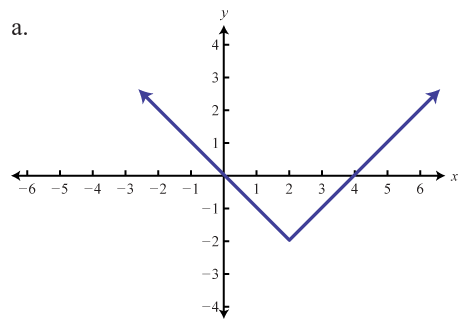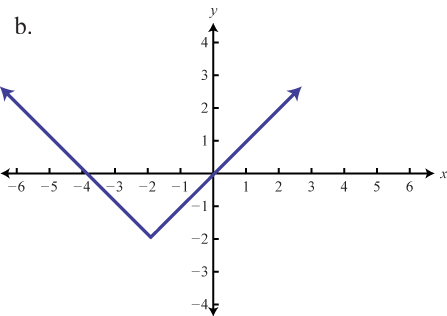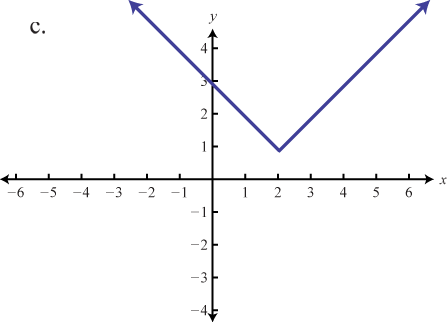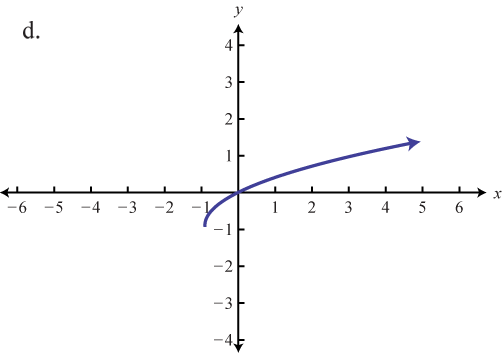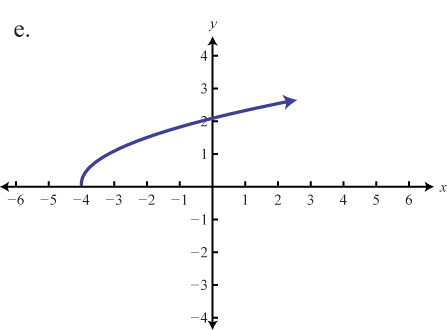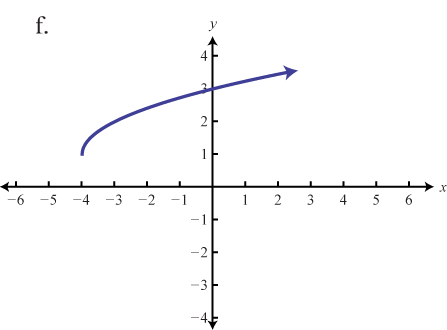1. $f(x)=x+4$

2. $f(x)=|x−2|−2$

3. $f(x)=x+1−1$

4. $f(x)=|x−2|+1$

5. $f(x)=x+4+1$

6. $f(x)=|x+2|−2$

Graph the given function. Identify the basic function and translations used to sketch the graph. Then state the domain and range.

1. $f(x)=x+3$

2. $f(x)=x−2$

3. $g(x)=x2+1$

4. $g(x)=x2−4$

5. $g(x)=(x−5)2$

6. $g(x)=(x+1)2$

7. $g(x)=(x−5)2+2$

8. $g(x)=(x+2)2−5$

9. $h(x)=|x+4|$

10. $h(x)=|x−4|$

11. $h(x)=|x−1|−3$

12. $h(x)=|x+2|−5$

13. $g(x)=x−5$

14. $g(x)=x−5$

15. $g(x)=x−2+1$

16. $g(x)=x+2+3$

17. $h(x)=(x−2)3$

18. $h(x)=x3+4$

19. $h(x)=(x−1)3−4$

20. $h(x)=(x+1)3+3$

21. $f(x)=1x−2$

22. $f(x)=1x+3$

23. $f(x)=1x+5$

24. $f(x)=1x−3$

25. $f(x)=1x+1−2$

26. $f(x)=1x−3+3$

27. $g(x)=−4$

28. $g(x)=2$

29. $f(x)=x−23+6$

30. $f(x)=x+83−4$

Graph the piecewise functions.

1. $h(x)={x2+2 if x<0x+2 if x≥0$
2. $h(x)={x2−3 if x<0x−3 if x≥0$
3. $h(x)={x3−1 if x<0|x−3|−4 if x≥0$
4. $h(x)={ x3ifx<0(x−1)2−1ifx≥0$
5. $h(x)={x2−1 if x<02 if x≥0$
6. $h(x)={x+2ifx<0(x−2)2ifx≥0$
7. $h(x)={(x+10)2−4 if x<−8x+4 if −8≤x<−4x+4 if x≥−4$
8. $f(x)={x+10 if x≤−10|x−5|−15 if −1020$

Write an equation that represents the function whose graph is given.

1.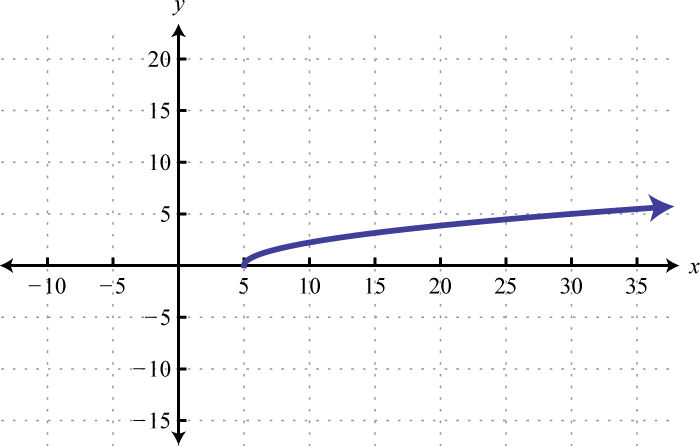2.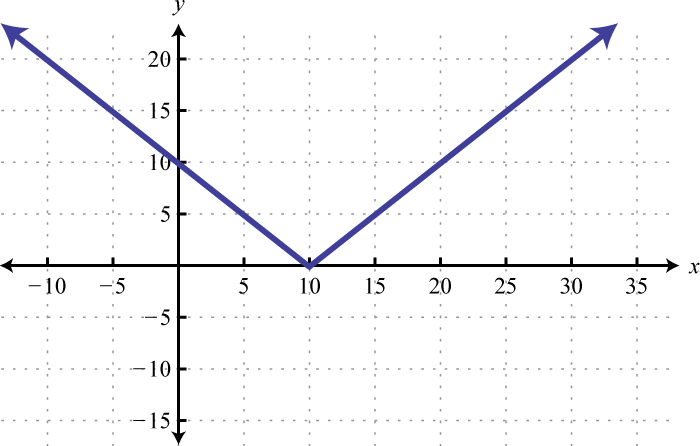3.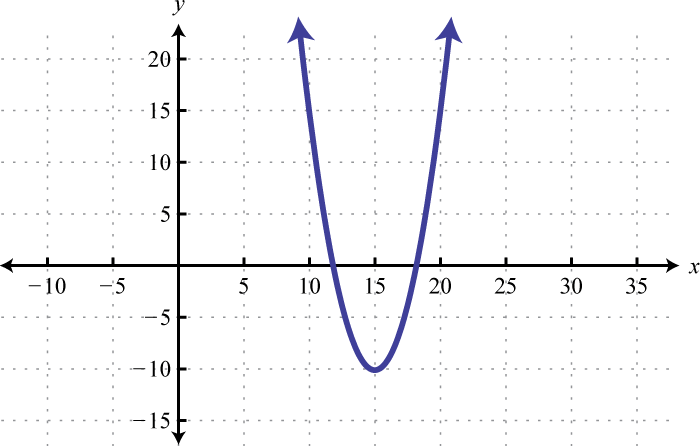4.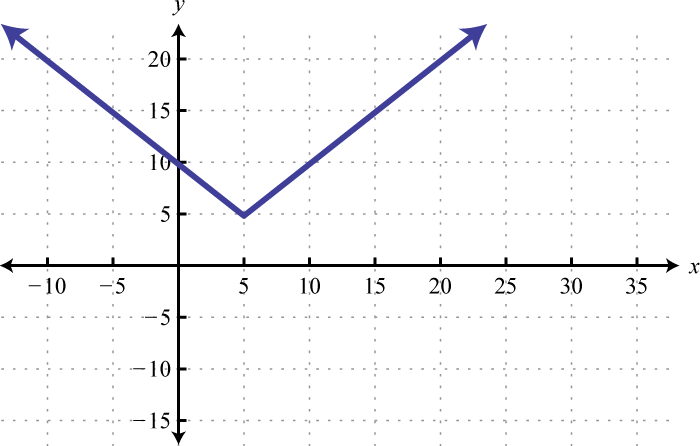5.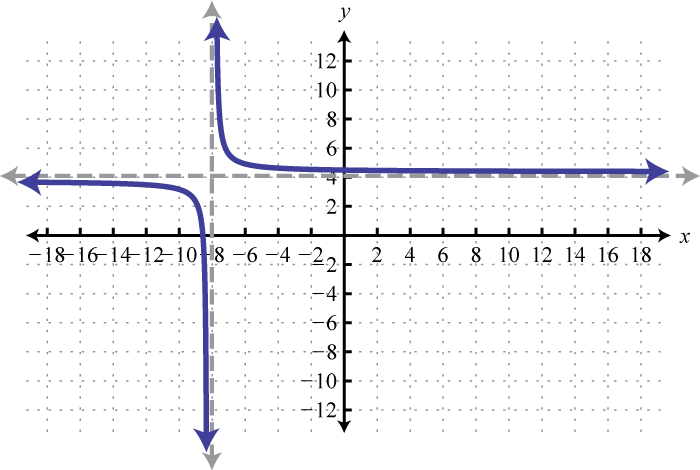6.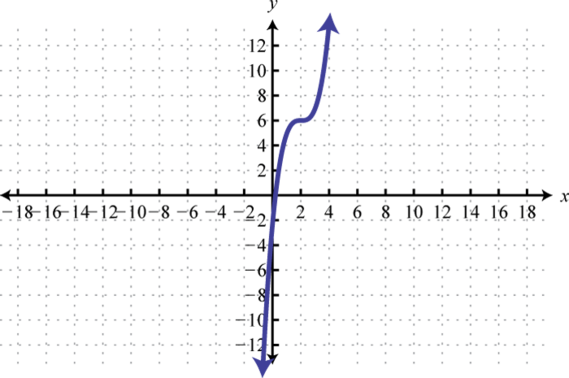7.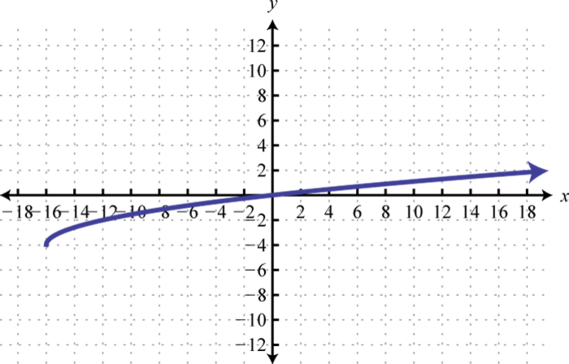8.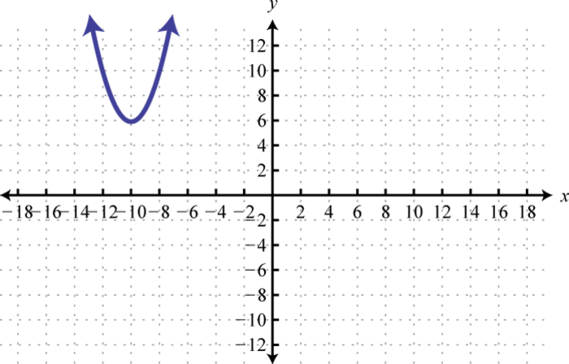### Part B: Reflections and Dilations

Match the graph the given function definition.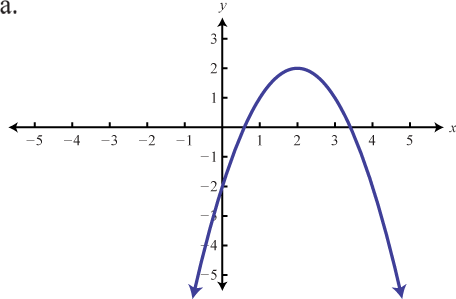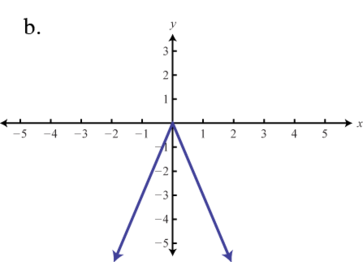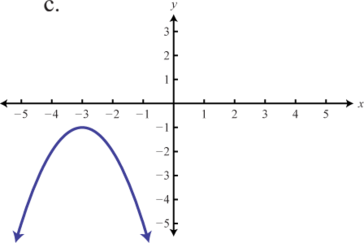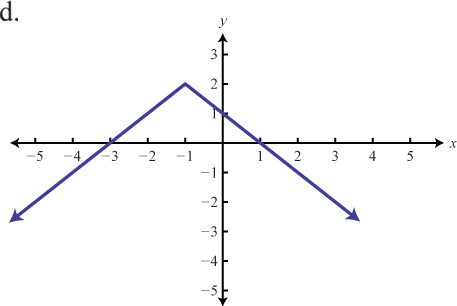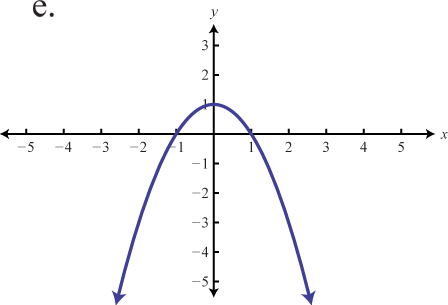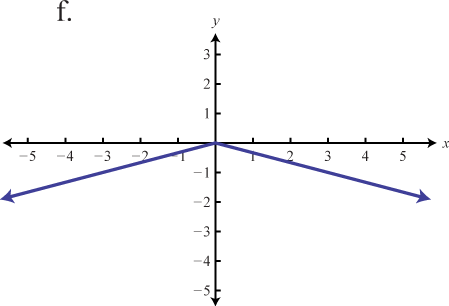1. $f(x)=−3|x|$

2. $f(x)=−(x+3)2−1$

3. $f(x)=−|x+1|+2$

4. $f(x)=−x2+1$

5. $f(x)=−13|x|$

6. $f(x)=−(x−2)2+2$

Use the transformations to graph the following functions.

1. $f(x)=−x+5$

2. $f(x)=−|x|−3$

3. $g(x)=−|x−1|$

4. $f(x)=−(x+2)2$

5. $h(x)=−x+2$

6. $g(x)=−x+2$

7. $g(x)=−(x+2)3$

8. $h(x)=−x−2+1$

9. $g(x)=−x3+4$

10. $f(x)=−x2+6$

11. $f(x)=−3|x|$

12. $g(x)=−2x2$

13. $h(x)=12(x−1)2$

14. $h(x)=13(x+2)2$

15. $g(x)=−12x−3$

16. $f(x)=−5x+2$

17. $f(x)=4x−1+2$

18. $h(x)=−2x+1$

19. $g(x)=−14(x+3)3−1$

20. $f(x)=−5(x−3)2+3$

21. $h(x)=−3|x+4|−2$

22. $f(x)=−1x$

23. $f(x)=−1x+2$

24. $f(x)=−1x+1+2$

### Part C: Discussion Board

1. Use different colors to graph the family of graphs defined by $y=kx2$, where $k∈{1,12,13,14}.$ What happens to the graph when the denominator of k is very large? Share your findings on the discussion board.

2. Graph $f(x)=x$ and $g(x)=−x$ on the same set of coordinate axes. What does the general shape look like? Try to find a single equation that describes the shape. Share your findings.

3. Explore what happens to the graph of a function when the domain values are multiplied by a factor a before the function is applied, $f(ax).$ Develop some rules for this situation and share them on the discussion board.

1. e

2. d

3. f

4.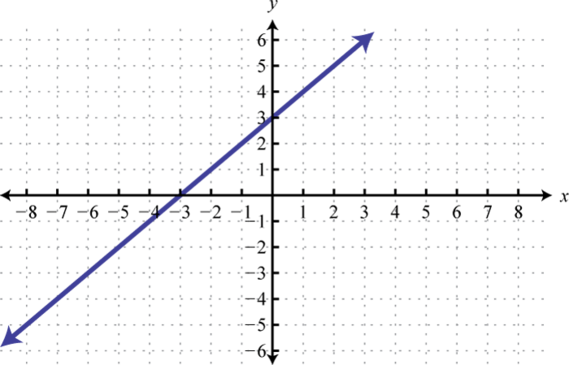$y=x$; Shift up 3 units; domain: $ℝ$; range: $ℝ$

5.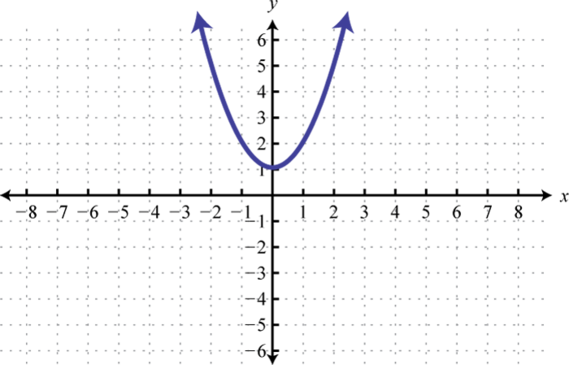$y=x2$; Shift up 1 unit; domain: $ℝ$; range: $[1,∞)$

6.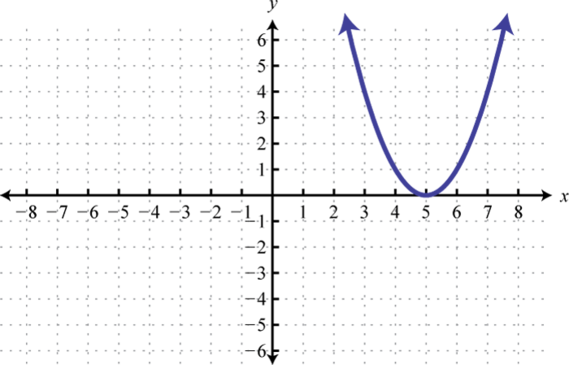$y=x2$; Shift right 5 units; domain: $ℝ$; range: $[0,∞)$

7.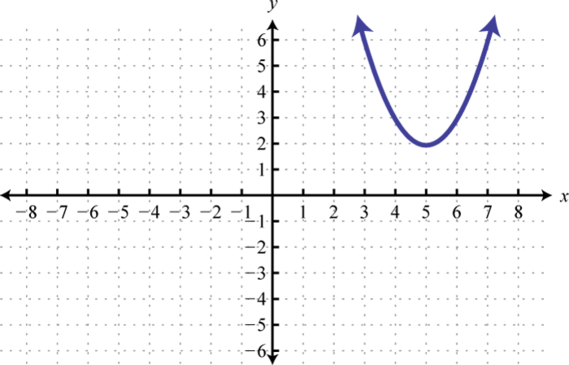$y=x2$; Shift right 5 units and up 2 units; domain: $ℝ$; range: $[2,∞)$

8.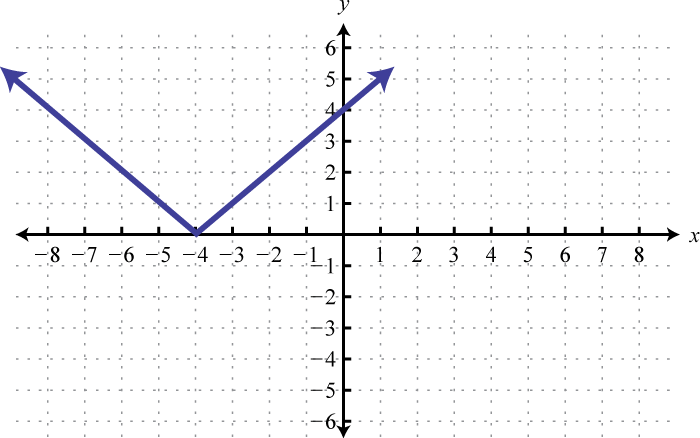$y=|x|$; Shift left 4 units; domain: $ℝ$; range: $[0,∞)$

9.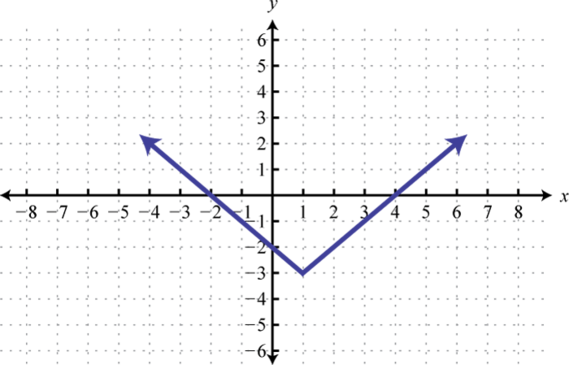$y=|x|$; Shift right 1 unit and down 3 units; domain: $ℝ$; range: $[−3,∞)$

10.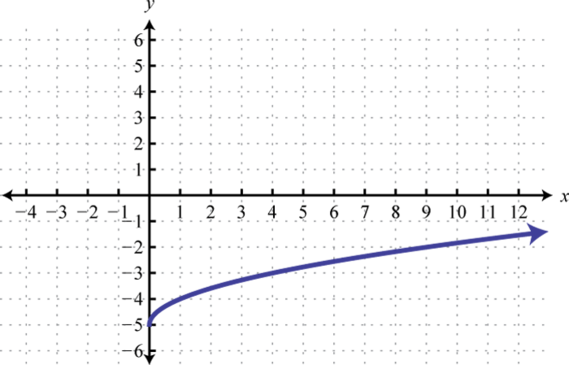$y=x$; Shift down 5 units; domain: $[0,∞)$; range: $[−5,∞)$

11.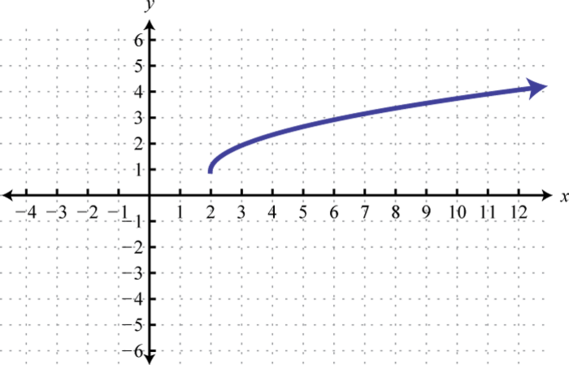$y=x$; Shift right 2 units and up 1 unit; domain: $[2,∞)$; range: $[1,∞)$

12.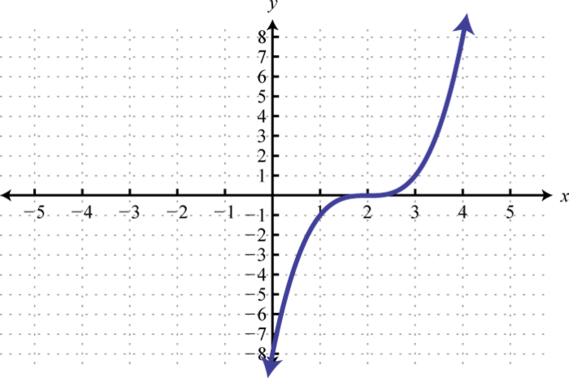$y=x3$; Shift right 2 units; domain: $ℝ$; range: $ℝ$

13.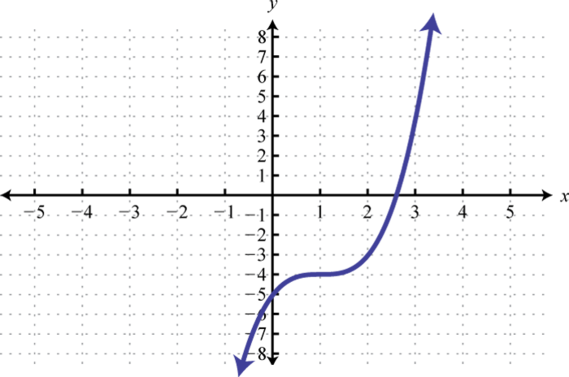$y=x3$; Shift right 1 unit and down 4 units; domain: $ℝ$; range: $ℝ$

14.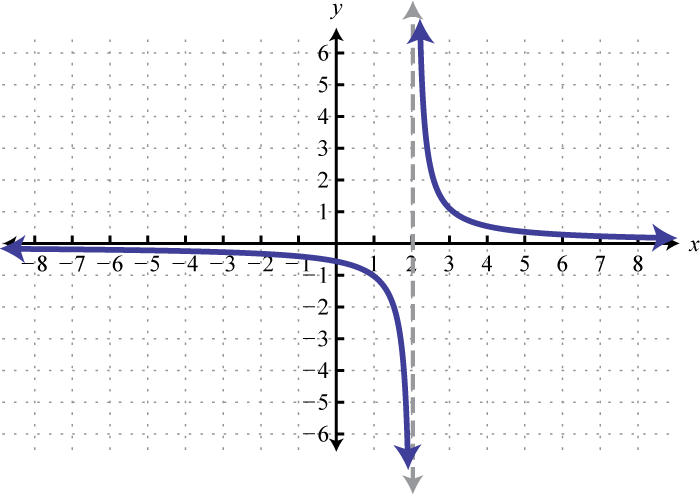$y=1x$; Shift right 2 units; domain: $(−∞,2)∪(2,∞)$; range: $(−∞,0)∪(0,∞)$

15.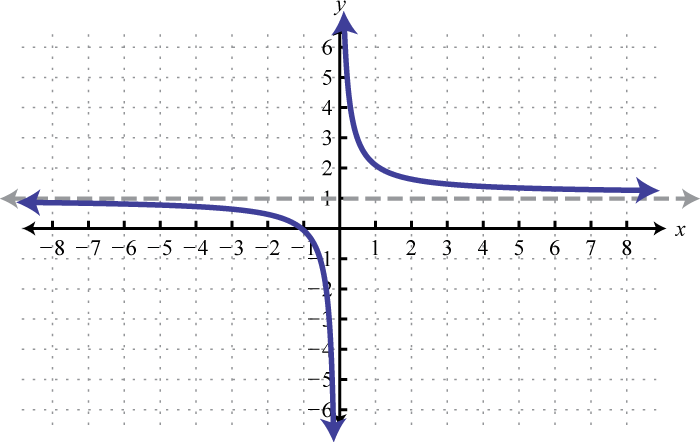$y=1x$; Shift up 5 units; domain: $(−∞,0)∪(0,∞)$; range: $(−∞,1)∪(1,∞)$

16.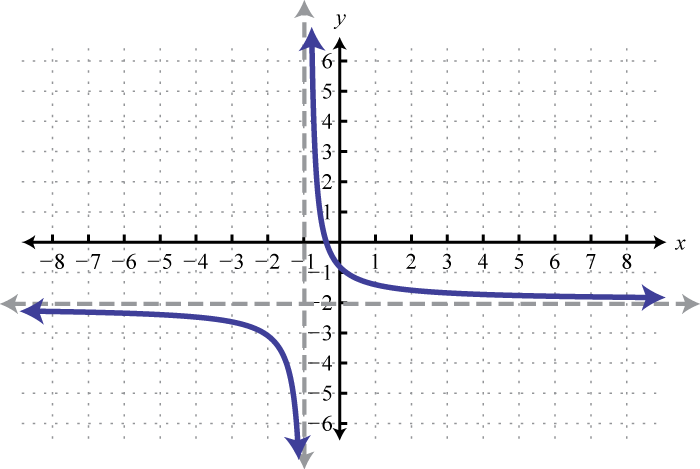$y=1x$; Shift left 1 unit and down 2 units; domain: $(−∞,−1)∪(−1,∞)$; range: $(−∞,−2)∪(−2,∞)$

17.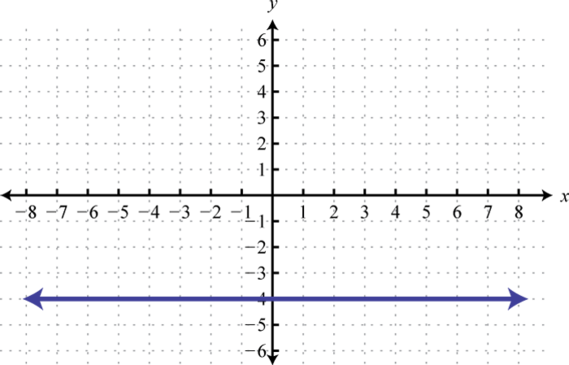Basic graph $y=−4$; domain: $ℝ$; range: {−4}

18.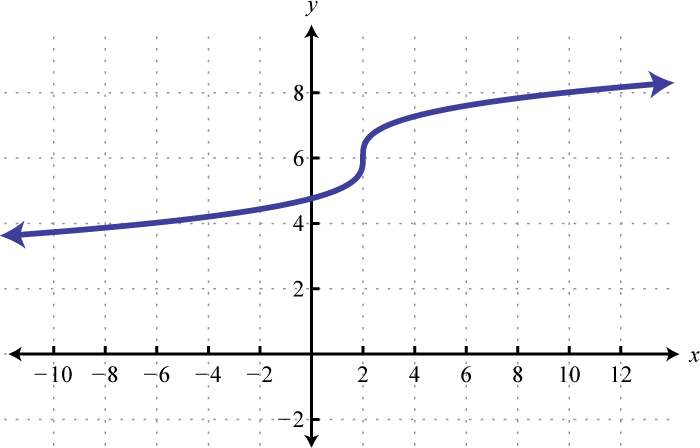$y=x3$; Shift up 6 units and right 2 units; domain: $ℝ$; range: $ℝ$

19.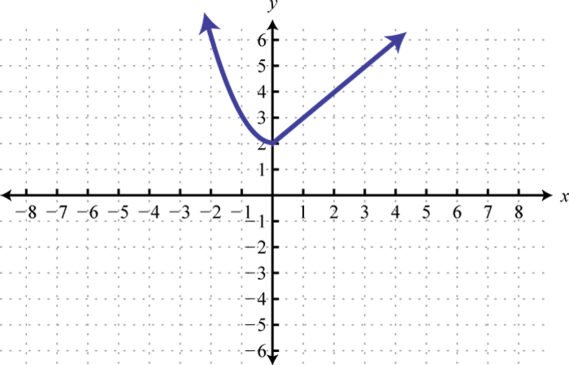20.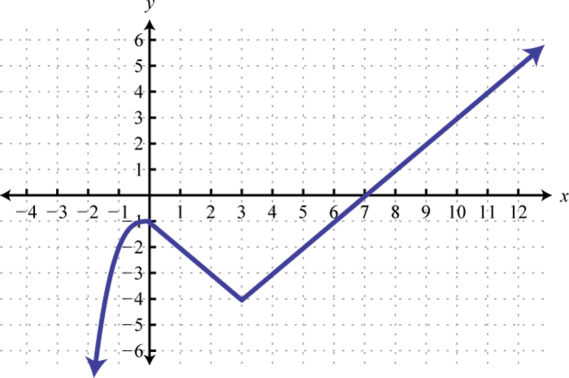21.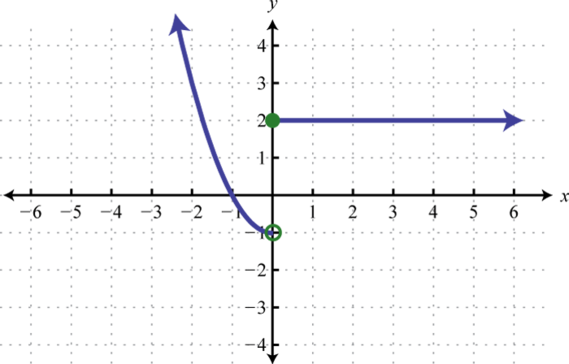22.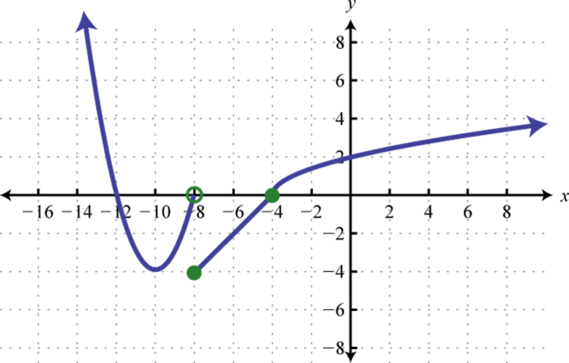23. $f(x)=x−5$

24. $f(x)=(x−15)2−10$

25. $f(x)=1x+8+4$

26. $f(x)=x+16−4$

1. b

2. d

3. f

4.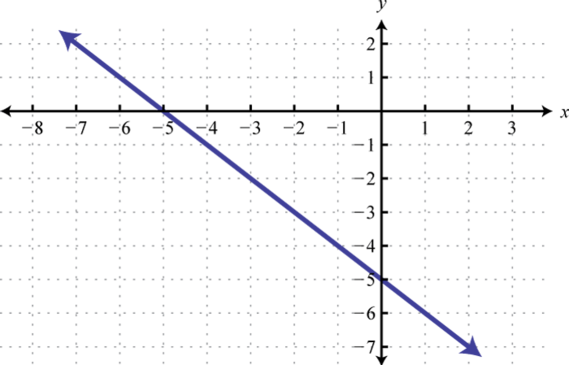5.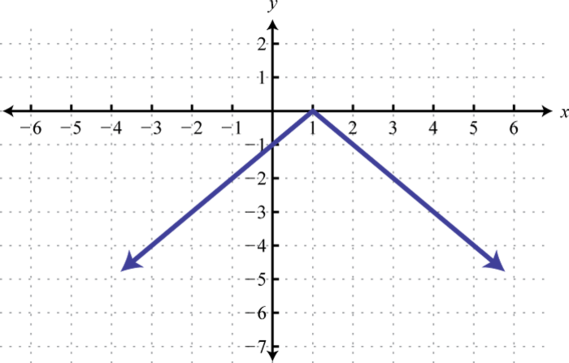6.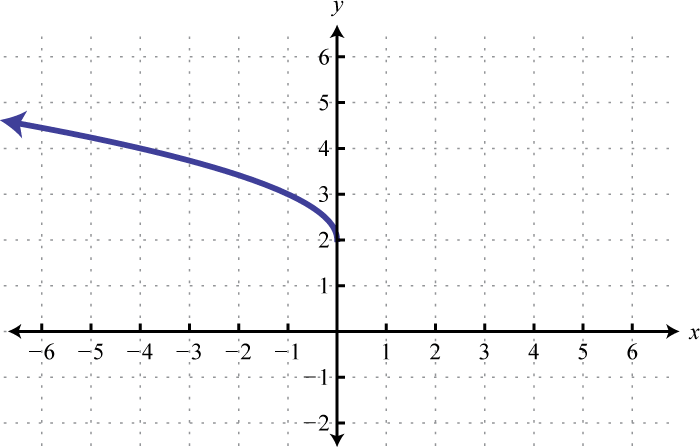7.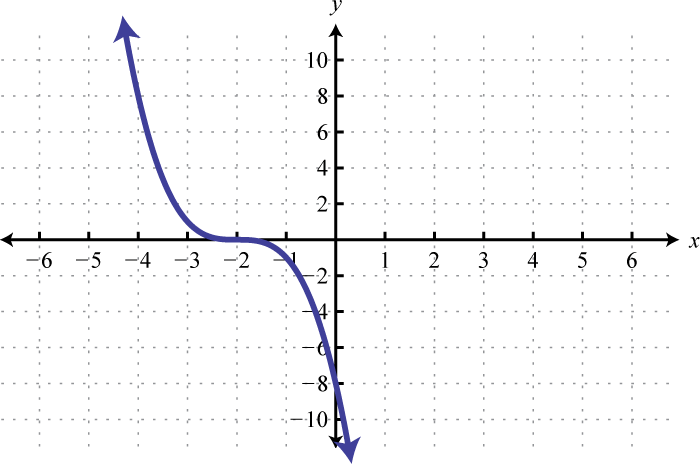8.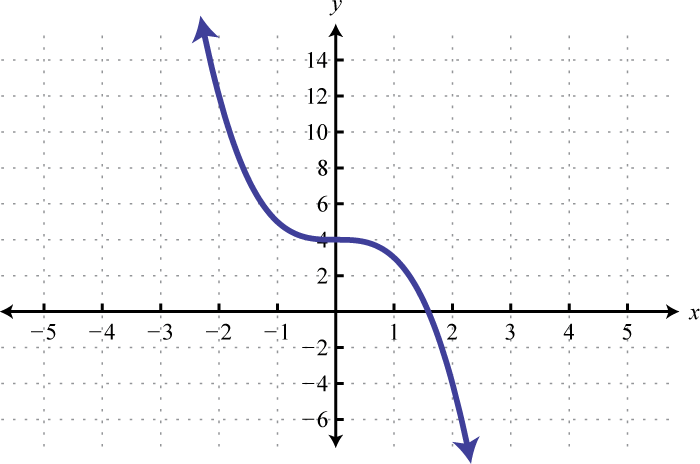9.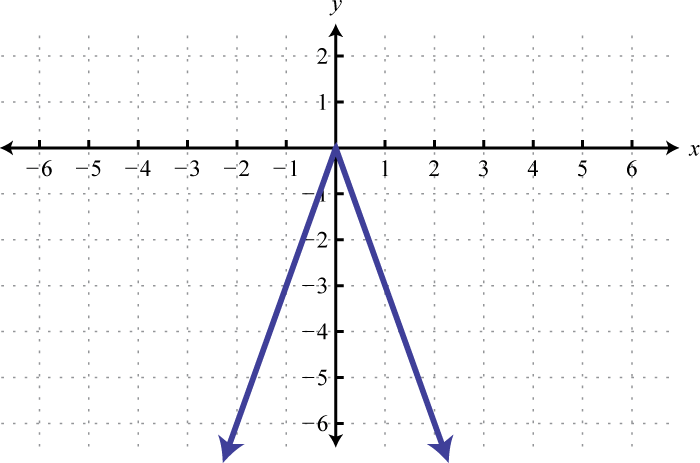10.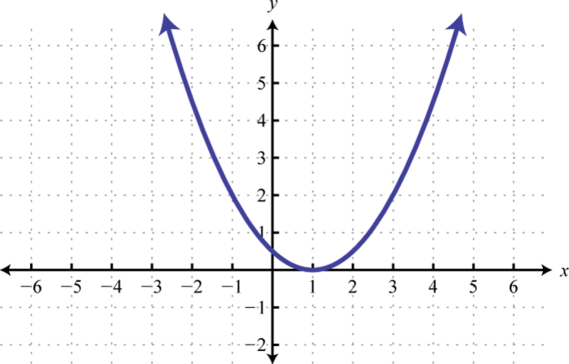11.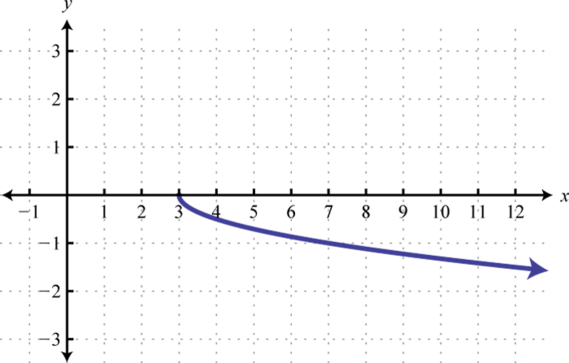12.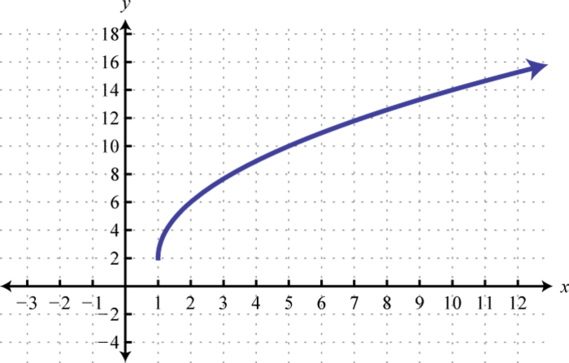13.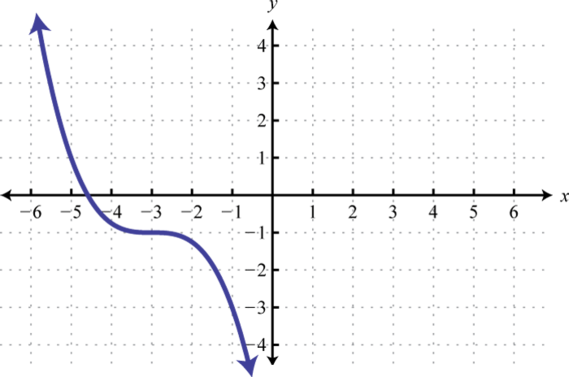14.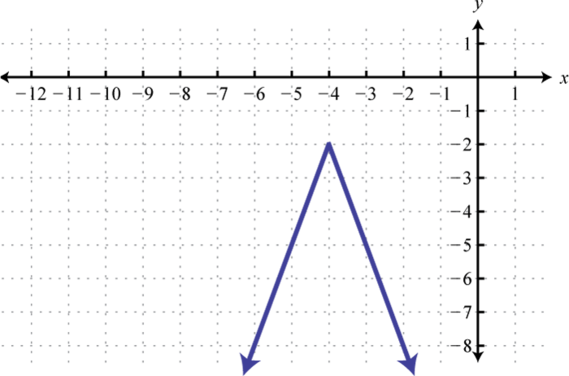15.